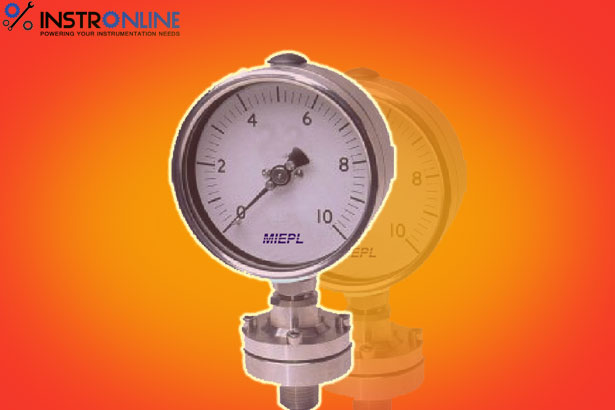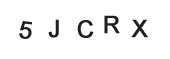• Home
• »
• Blog
• »
• Basics of Pressure Measurement At Instronline Instrumentation
Discussion Forum

##### Basics of Pressure Measurement At Instronline Instrumentation
Listed on Aug 28,2018A major portion of all industrial measurement relates in some way to pressure in its several forms. Flow, for example, is often measured by determining the pressure that exists at two different points in a system. In a Bourdon system, pressure changes are used to produce the mechanical motion of a recording stylus.

Pressure can also be used to measure temperature in a filled system through changes produces by an expansion of the liquid or fluid in the filled system.

Pressure measurement is made more than any measurement in the process industries. It is the best quick indication of the work done by pumps and compressors. It is also the most important measure of the status of operating pressure vessels.

What is Pressure ?

For the pressure in a container, it is a measure of force exerted by a fluid due to its molecular activities. This is a derivation from the concept above combined with the Kinetic Theory of Matters.

Types of Pressure Exerted by Fluids

Pressure Produced by Liquids

All fluids (liquids and gases) have weight, thus they exert a pressure against the wall of their containers. The pressure exerted by a liquid at any given point or location in the vessel depends upon the height of liquid above it. This pressure is independent of the shape of the vessel.

Pressure Produced by Density

Density is the weight of one cubic meter of material. Different liquids weight different amounts for the same volume and therefore would create different pressures. Since we know that, pressure is equal to force (or weight) over a unit area, and in any fixed volume of column, the weight of liquid contained varies with density; therefore density change will change the pressure of the container.

Pressure Produced by Gases

To study some of the principles governing the behaviour of gases, we will use air as common gas known. Since air does have weight, it builds up static pressure, much the same as liquids do. One cubic foot of air weighs about 0.08 lbs. A room 10 feet wide, 20 feel long and 8 feet high has 1600 cubic feet of air in it weighing about 128 lbs. The deep layer of air which blankets the earth exerts a pressure much like the water pressure at the bottom of the ocean. This pressure is known as atmospheric pressure and is about 14.7 psi at sea level.

The final or total pressure exerted at any given point in a vessel, pump, line, etc. will depend upon the head, or weight of liquid being handled plus any external pressure being exerted on the liquid being handled. Figure below shows the effect of the external pressure.

Vessel filled with water to a height 20 feet and is open to atmosphere. Pressure at the bottom would be 20 × 0.433, or 8.66 psi. If we close the vessel and add compressed gas of 200 psi on the top of the vessel, we would find that pressure of the bottom would be (200 + 8.66) psi, or 208.66 psi.

Glossary of Pressure
Two reference points for pressure measurement exists. The most logical one is absolute zero – a condition existing only in a perfect vacuum. Pressures measured from this reference point are called absolute pressure.

The other reference point used is atmospheric pressure. The difficulty with this reference point is that it changes with altitude (reference with sea level) and to some extent with weather conditions. At or near sea level, this pressure is about 14.7 psia or 29.9 inches or 760 mmHga.

Absolute Pressure, Pabs

Absolute pressure is the pressure measured with respect to zero pressure (vacuum).

Gauge Pressure, Pgauge

Gauge pressure (or sometimes called Internal pressure) is the pressure measured by a gauge in access of the pressure of the atmosphere. A pressure gauge indicates the difference between pressure on a system or vessel and the local atmospheric pressure. However, be careful ! when reading a pressure gauge; determine whether it is reading absolute pressure or gauge pressure, normally indicated by “a” or “g”, e.g. psia or barg.

Atmospheric Pressure, Patm

Atmospheric pressure is the pressure measure on the surface in the atmosphere. However, the difficulty is that it changes with altitude and humidity. Thus, atmospheric pressure may differ from one area or place to another.

Differential Pressure (DP)

Differential pressure is the pressure difference, measured between two different pressure points, within the same pressure reference point., e.g. between unknown pressure and a local atmospheric pressure.

Head pressure is the pressure exerted by a liquid that indicated by height of the liquid in a liquid column.

It depends on;

1. the height of the liquid column.

2. the relative density of the liquid.

Pressure head = R.D x h x 9.8 kPa

where, R.D = relative density of the liquid,  h = the height of the liquid g = gravitational force

Note: Relative density is the density of a liquid relative to the referral standard density (water = 1000 kgm-3)

Vacuum

Pressure less than atmospheric pressure is called vacuum. A vacuum is a lack of air fluid. The vacuum scale extend between the absolute zero reference point and atmospheric pressure, thus it is not a positive pressure. It is treated as a sucking force or negative pressure.

Pressure Conversions between Units

Methods of Pressure Measurement

There are 3 basic methods for pressure measurement;

I. The first method involves balancing the unknown pressure against the pressure produced by a column of liquid of known density.

II. The second method involves allowing the unknown pressure to act on a known area and measuring the resultant force either directly or indirectly.

III. The third method involves allowing the unknown pressure to act on an elastic material and measuring the resultant stress or strain.Tagged Popular Searches

Related Search